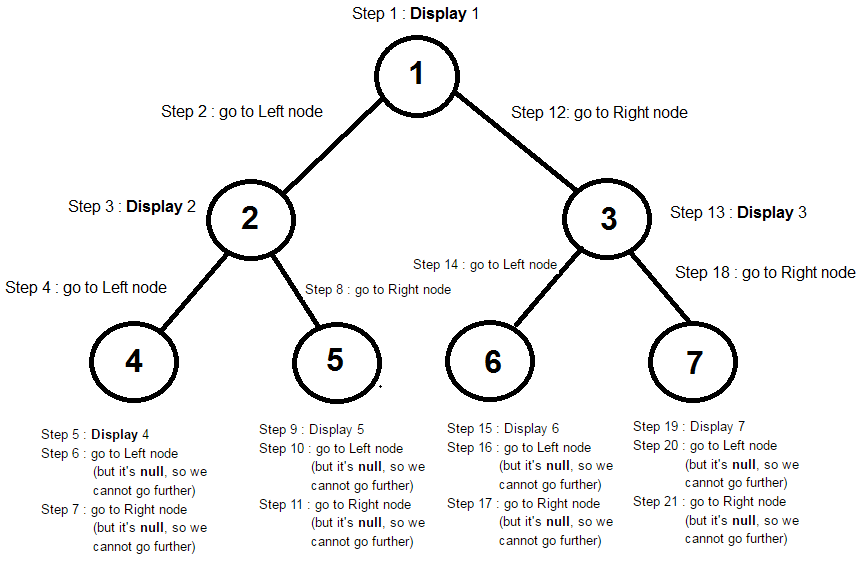### PreOrder traversal of Binary Tree in java

In this data structure tutorial we will learn PreOrder traversal of Binary Tree in java with program and examples.

Contents of page >
1. What happens in PreOrder traversal of Binary Tree in java >
2. What are Steps in PreOrder Traversal of Binary Tree in java >
3. Diagram of PreOrder traversal of Binary Tree in java >
Result of PreOrder traversal of Binary Tree in java is >
1. Logic for PreOrder traversal of Binary Tree in java >
2. Full program/ example to show PreOrder traversal of Binary Tree in java >

1. What happens in PreOrder traversal of Binary Tree in java >
In PreOrder traversal of Binary Tree in java each node is processed before traversing its subtree.

2. What are Steps in PreOrder Traversal of Binary Tree in java >
Steps in PreOrder Traversal of Binary Tree in java -
1. Visit the node.
2. Traverse the left subtree.
3. Traverse the right subtree.

Diagram of PreOrder traversal of Binary Tree in java >Result of PreOrder traversal of Binary Tree in java is >
1 2 4 5 3 6 7

4. Logic for PreOrder traversal of Binary Tree in java >
 /**    * This method will do pre-order Traversal of binary tree in java.    * Method will pass leftNode of binary tree recursively    * Once leftNode child is done, pass rightNode of binary tree recursively    */    public void preorderTraversalMethod(BinaryTreeNode binaryTreeNode) {           // Continue if binaryTreeNode is not null           if (binaryTreeNode != null) {                  // Display data of current binaryTreeNode.                  System.out.print(binaryTreeNode.data + " ");                  // pass leftNode of binary tree recursively                  preorderTraversalMethod(binaryTreeNode.leftNode);                  // pass rightNode of binary tree recursively                  preorderTraversalMethod(binaryTreeNode.rightNode);           }    }

5. Full program/ example to show PreOrder traversal of Binary Tree in java >
 /** * www.JavaMadeSoEasy.com * Write a program to perform PreOrder Traversal of Binary Tree in java * * Class to perform PreOrder Traversal of Binary Tree in java */ public class PreOrderTraversalOfBinaryTree {       /**    * Create Node of Binary Tree    */    public static class BinaryTreeNode {           // Left and right node of Binary tree           BinaryTreeNode leftNode;           BinaryTreeNode rightNode;           // Data of BinaryTree           int data;           BinaryTreeNode(int data) {                  this.data = data;           }    }    /**    * This method will do pre-order Traversal of binary tree in java.    * Method will pass leftNode of binary tree recursively    * Once leftNode child is done, pass rightNode of binary tree recursively    */    public void preorderTraversalMethod(BinaryTreeNode binaryTreeNode) {           // Continue if binaryTreeNode is not null           if (binaryTreeNode != null) {                  // Display data of current binaryTreeNode.                  System.out.print(binaryTreeNode.data + " ");                  // pass leftNode of binary tree recursively                  preorderTraversalMethod(binaryTreeNode.leftNode);                  // pass rightNode of binary tree recursively                  preorderTraversalMethod(binaryTreeNode.rightNode);           }    }    /**    * Main method to perform PreOrder Traversal of Binary Tree in java    */    public static void main(String[] args) {           PreOrderTraversalOfBinaryTree preOrderTraversalOfBinaryTree =                                             new PreOrderTraversalOfBinaryTree();           // Now, let's start creating nodes of Binary tree           // create node1 (which is ROOT node)           BinaryTreeNode binaryTreeNode1 = new BinaryTreeNode(1);           // Left node of node1           BinaryTreeNode node2 = new BinaryTreeNode(2);           binaryTreeNode1.leftNode = node2;           // Right node of node1           BinaryTreeNode node3 = new BinaryTreeNode(3);           binaryTreeNode1.rightNode = node3;           // Left node of node2           BinaryTreeNode node4 = new BinaryTreeNode(4);           node2.leftNode = node4;           // Right node of node2           BinaryTreeNode node5 = new BinaryTreeNode(5);           node2.rightNode = node5;           // Left node of node3           BinaryTreeNode node6 = new BinaryTreeNode(6);           node3.leftNode = node6;           // Right node of node3           BinaryTreeNode node7 = new BinaryTreeNode(7);           node3.rightNode = node7;           System.out.println("Pre order Traversal of Binary Tree in java : ");           // Pass root node to pre order traversal method           preOrderTraversalOfBinaryTree.preorderTraversalMethod(binaryTreeNode1);    } } /* Output Pre order Traversal of Binary Tree in java : 1 2 4 5 3 6 7 */

Summary -
So in this data structure tutorial we learned In PreOrder traversal of Binary Tree in java each node is processed before traversing its subtree. Steps in PreOrder Traversal of Binary Tree in java. Diagram of PreOrder traversal of Binary Tree in java.Logic for PreOrder traversal of Binary Tree in java. Full program/ example to show PreOrder traversal of Binary Tree in java.

Having any doubt? or you liked the tutorial! Please comment in below section.